# Searching Arrays Linear search small arrays unsorted arrays

• Slides: 15
Download presentation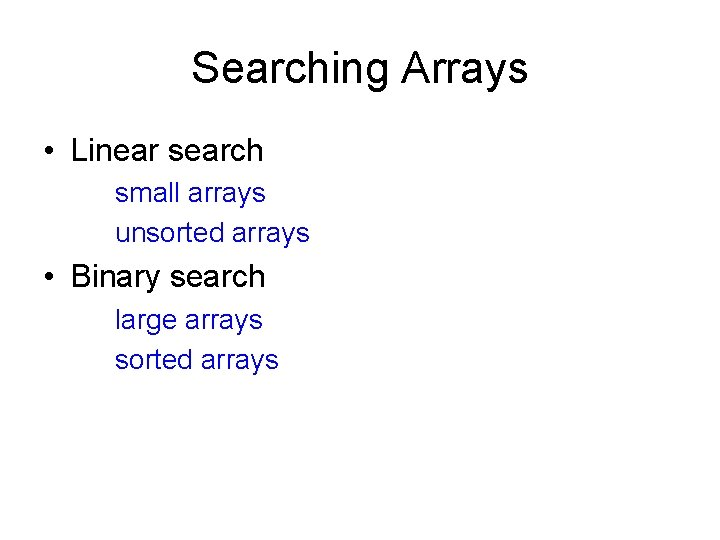Searching Arrays • Linear search small arrays unsorted arrays • Binary search large arrays sorted arraysLinear Search Algorithm Start at first element of array. Compare value to value (key) for which you are searching Continue with next element of the array until you find a match or reach the last element in the array. Note: On the average you will have to compare the search key with half the elements in the array.Linear Search Code const int array. Size = 100; int a[array. Size] = {1, 100, 2, 66, 55, 44, 88, 77, 12, 23, 45, 9, 87}; int key = 88; bool found = false; for (int i = 0; i < array. Size; i++) { if (a[i] == key) { cout << “Found it at array subscript “ << i << endl; found = true; break; } } if (! found) cout << “Could not find element “ << key << “ in array a” << endl;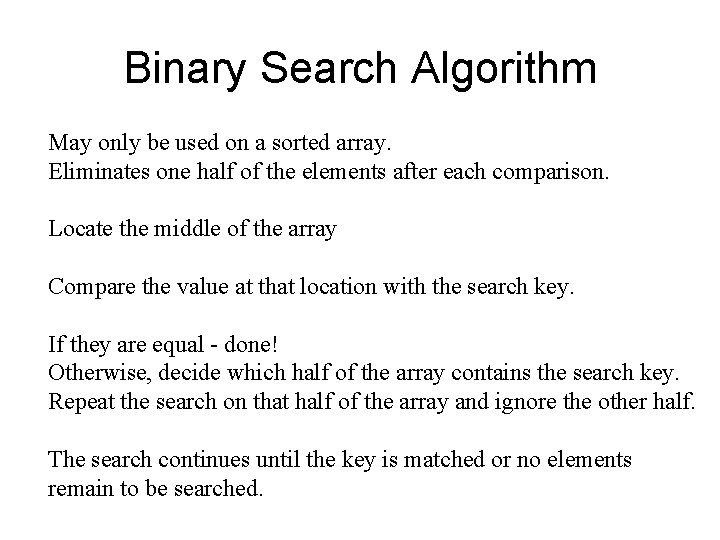Binary Search Algorithm May only be used on a sorted array. Eliminates one half of the elements after each comparison. Locate the middle of the array Compare the value at that location with the search key. If they are equal - done! Otherwise, decide which half of the array contains the search key. Repeat the search on that half of the array and ignore the other half. The search continues until the key is matched or no elements remain to be searched.Binary Search Example a 0 1 2 3 4 5 6 7 8 9 10 11 12 1 5 15 19 25 27 29 31 33 45 55 88 100 search key = 19 middle of the array compare a and 19 19 is smaller than 29 so the next search will use the lower half of the array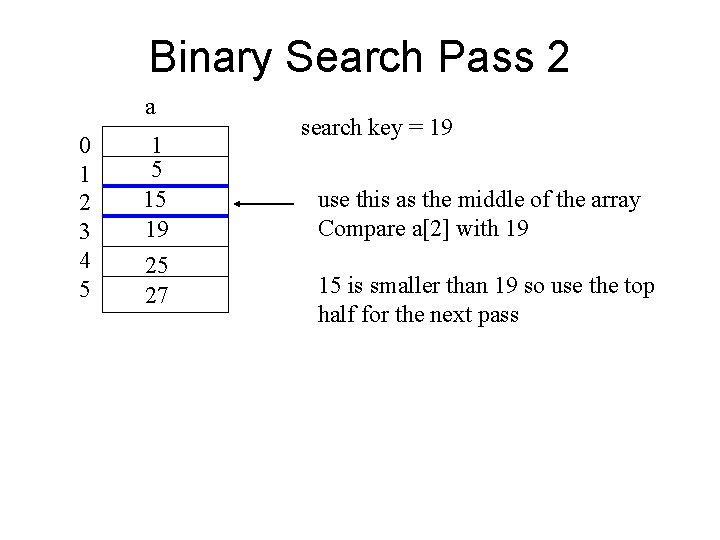Binary Search Pass 2 a 0 1 2 3 4 5 15 19 25 27 search key = 19 use this as the middle of the array Compare a with 19 15 is smaller than 19 so use the top half for the next pass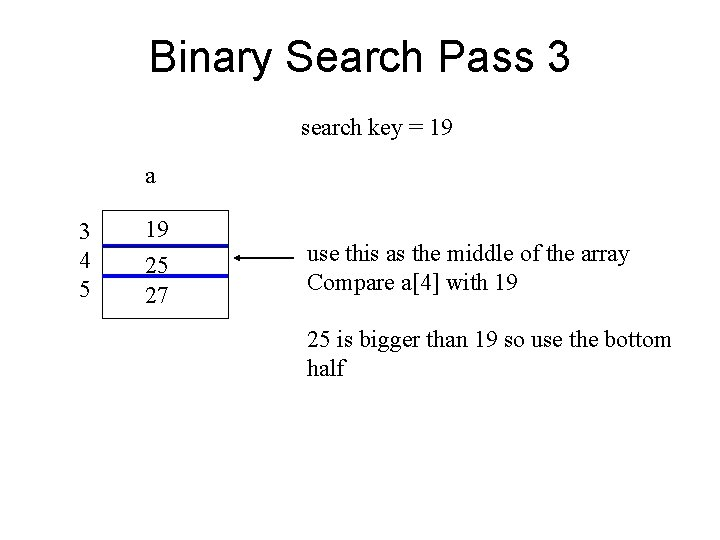Binary Search Pass 3 search key = 19 a 3 4 5 19 25 27 use this as the middle of the array Compare a with 19 25 is bigger than 19 so use the bottom half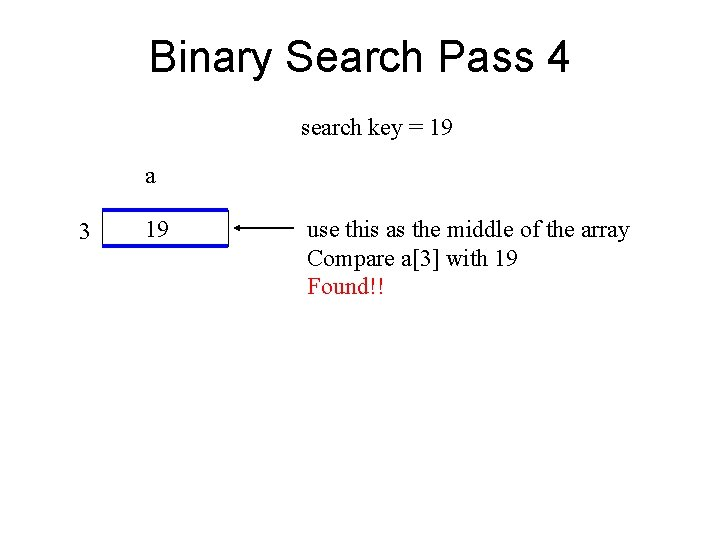Binary Search Pass 4 search key = 19 a 3 19 use this as the middle of the array Compare a with 19 Found!!Binary Search Example a 0 1 2 3 4 5 6 7 8 9 10 11 12 1 5 15 19 25 27 29 31 33 45 55 88 100 search key = 18 middle of the array compare a and 18 18 is smaller than 29 so the next search will use the lower half of the array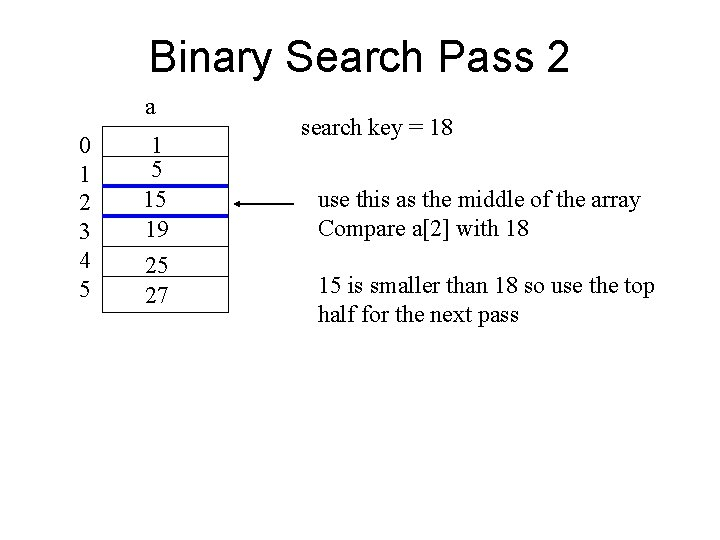Binary Search Pass 2 a 0 1 2 3 4 5 15 19 25 27 search key = 18 use this as the middle of the array Compare a with 18 15 is smaller than 18 so use the top half for the next pass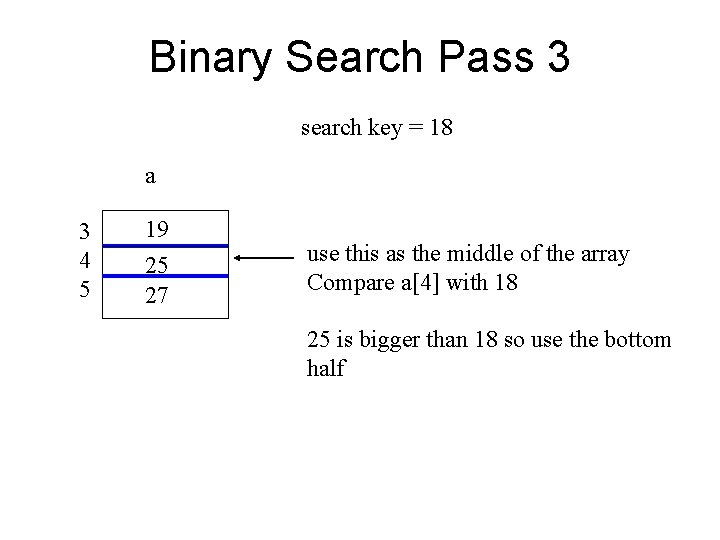Binary Search Pass 3 search key = 18 a 3 4 5 19 25 27 use this as the middle of the array Compare a with 18 25 is bigger than 18 so use the bottom halfBinary Search Pass 4 search key = 18 a 3 19 use this as the middle of the array Compare a with 18 Does not match and no more elements to compare. Not Found!!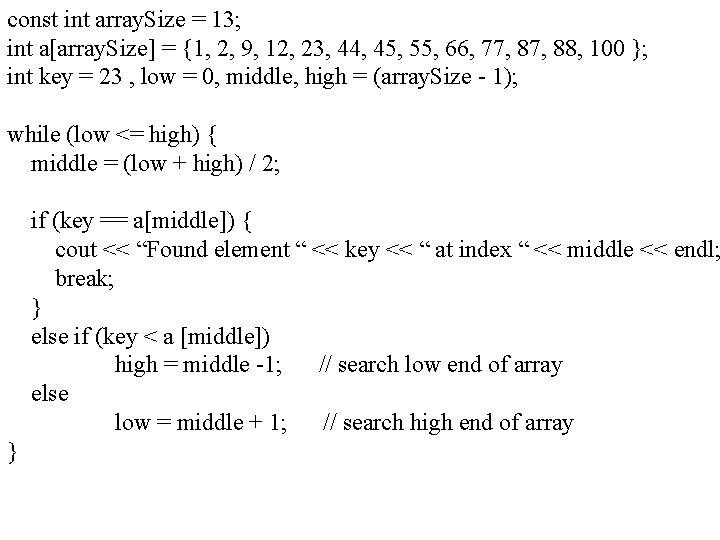const int array. Size = 13; int a[array. Size] = {1, 2, 9, 12, 23, 44, 45, 55, 66, 77, 88, 100 }; int key = 23 , low = 0, middle, high = (array. Size - 1); while (low <= high) { middle = (low + high) / 2; if (key == a[middle]) { cout << “Found element “ << key << “ at index “ << middle << endl; break; } else if (key < a [middle]) high = middle -1; // search low end of array else low = middle + 1; // search high end of array }Efficiency Searching and array of 1024 elements will take at most 10 passes to find a match or determine that the element does not exist in the array. 512, 256, 128, 64, 32, 16, 8, 4, 2, 1 An array of one billion elements takes a maximum of 30 comparisons. The bigger the array the better a binary search is as compared to a linear search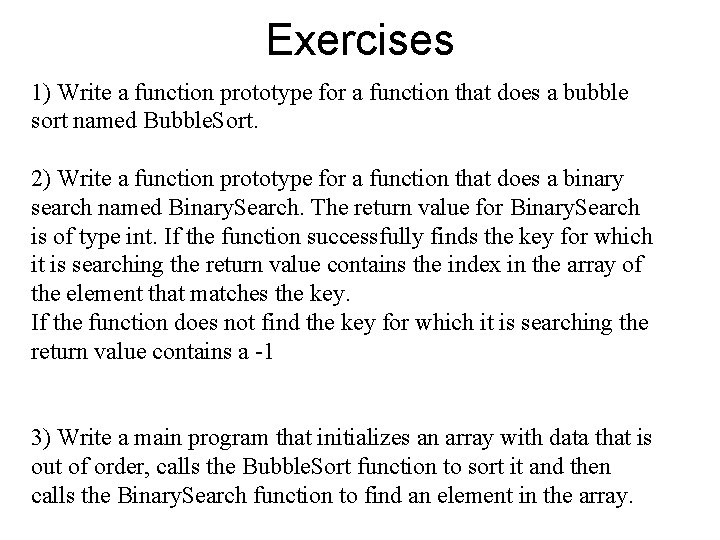Exercises 1) Write a function prototype for a function that does a bubble sort named Bubble. Sort. 2) Write a function prototype for a function that does a binary search named Binary. Search. The return value for Binary. Search is of type int. If the function successfully finds the key for which it is searching the return value contains the index in the array of the element that matches the key. If the function does not find the key for which it is searching the return value contains a -1 3) Write a main program that initializes an array with data that is out of order, calls the Bubble. Sort function to sort it and then calls the Binary. Search function to find an element in the array.